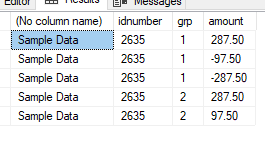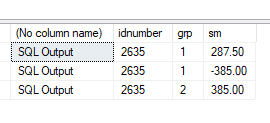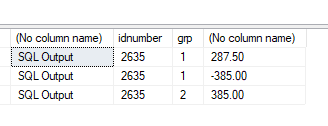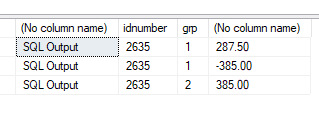# Identify the corresponding negative number and separate left over positive number

example 1
idnumber grp amount
2635 1 287.50
2635 1 -97.50
2635 1 -287.50
2635 2 287.50
2635 2 97.50

expected output

idnumber grp amount
2635 1 287.50
2635 1 -385
2635 1 385

hi

``````create table sample_data
(
idnumber int
, grp int
, amount decimal(10,2)
)
go

insert into sample_data values
(2635, 1 ,287.50),
(2635, 1 ,-97.50),
(2635, 1 ,-287.50),
(2635, 2 ,287.50),
(2635, 2, 97.50)

select * from
(
select idnumber,grp,sum(case when amount > 0 then amount else 0 end )  as sm from sample_data
group by idnumber,grp
union all
select idnumber,grp,sum(case when amount < 0 then amount else 0 end )  as sm from sample_data
group by idnumber,grp
) a
where a.sm <> 0
order by 1,2,sm desc
``````1 Like

hi

another way to do this ..

which approach to do ... why ???? also other approaches .. Performance Tuning Reasons
Logical Reads , Time Taken etc are there ..dealing with large amounts of data
are also there !!

``````; with cte as
(
select case when amount > 0 then 1 else 2 end as neg_pos , * from sample_data
)
select
distinct  idnumber , grp , sum(amount) over(partition by idnumber,grp,neg_pos  order by idnumber,grp )
from
cte
order by
1,2,3 desc
``````hi

another way to do this !!

``````; with cte as
(
select
case when amount > 0 then 1 else 2 end as neg_pos
, *
from
sample_data
)
select
idnumber
, grp
, sum(amount)
from
cte
group by
idnumber
, grp
, neg_pos
``````2 Likes

Thank you, query works.